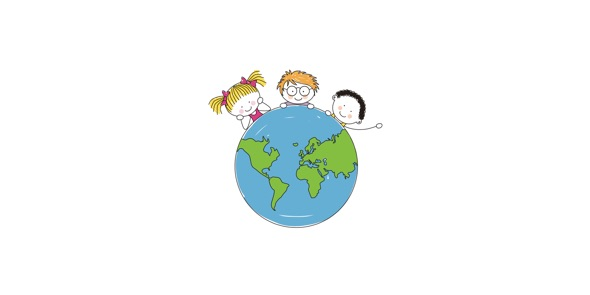# Take This Quiz On Introductory Unit Review!

11 Questions | Total Attempts: 66SettingsThe quiz reviews concepts learned in the 7th-grade. These concepts include measurement, scientific method, observation, and the proper care and usage of scientific instruments. So, let's try out the quiz. All the best!

• 1.
Which tool is used to measure the volume accurately?
• A.

Beaker

• B.

• C.

Triple-beam balance

• D.

Meter stick

• 2.
What is the basic unit of measurement for measuring the volume of a solid?
• A.

Meter

• B.

Liter

• C.

Cubic centimeter

• D.

Gram

• 3.
3.2 centimeters equals ____________ millimeters
• A.

.0032

• B.

.32

• C.

320

• D.

32

• 4.
.89 kimometers equals ____________ meters
• A.

.0089

• B.

8.9

• C.

890

• D.

89

• 5.
What is the first step in the scientific method?
• A.

Form a hypothesis

• B.

Identify the problem

• C.

Record the results

• D.

Conduct an experiment

• 6.
After coming up with a hypothesis, a good scientist must
• A.

Test the hypothesis by an experiment

• B.

Research the topic

• C.

Come to a conclusion

• D.

Collect and record the data

• 7.
The part of the experiment that is changed by the person performing the experiment is the
• A.

Controlled variable

• B.

Independent variable

• C.

Dependent variable

• D.

Invisible variable

• 8.
The part of the experiment that is measured (it is affected by the part of the experiment that is changed) is called the
• A.

Controlled variable

• B.

Independent variable

• C.

Dependent variable

• D.

Invisible variable

• 9.
What unit of measurement would you use to measure the length of a soccer field?
• A.

Gram

• B.

Centimeter

• C.

Kilometer

• D.

Meter

• 10.
Which of the following is NOT a unit of measurement?
• A.

Temperature

• B.

Length

• C.

Mass

• D.

None of the above

• 11.
Temperature is one unit of measurement. Which tool is used to measure temperature?
• A.

Scale

• B.

Thermometer

• C.

Pound

• D.

Hammer

Related TopicsBack to top